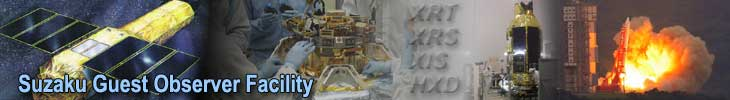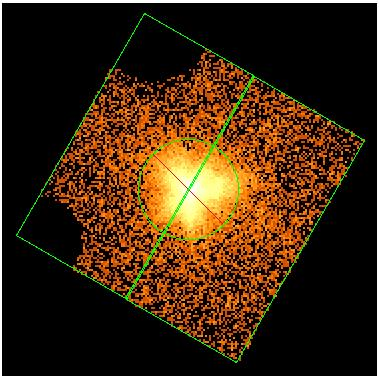## XISSIMARFGEN: Arf of Uniformly Extended Source

Here, we show an example of generating an ARF file for a uniformly extended source, using observtion 102002010. The following is an XIS0 image of the observation, in which the strong emission from SNR E0102.2-7219 is evident. Here, we try to search for possible extended emission from the surrounding areas. In this analysis, we screened out the calibration source so as not to degrade the data quality significantly. To do so, type

```XSEL> select events "(STATUS<524287)&&(STATUS%(2**17)<2**16)"
```

in XSELECT.As you see in this image, events at the two corners, where the calibration sources are located, have been removed. Here, we extract two spectra from the top-left half and bottom-right half of this image, whose region files are described by files e0102_tophalf_phys.reg, and e0102_bottomhalf_phys.reg.

Then, the appropriate ARFs can be generated using the following command.

xissimarfgen clobber=yes \
instrume=XIS0 \
pointing=AUTO \
source_mode=UNIFORM \
source_rmin=0 \
source_rmax=20 \
num_region=2 \
region_mode=SKYREG \
regfile1=e0102_tophalf_phys.reg \
regfile2=e0102_bottomhalf_phys.reg \
arffile1=e0102_tophalf.arf \
arffile2=e0102_bottomhalf.arf \
limit_mode=MIXED \
num_photon=2000000 \
accuracy=0.005 \
phafile=e0102_tophalf.pi \
gtifile=../xis/ae102020010xi0_cl.evt \
attitude=../auxil/ae102020010.att
\
rmffile=e0102_tophalf.rmf \
estepfile=medium

As in Example 1, options in black specify calibration files or Monte-Carlo simulation parameters that can be adjusted for each run of xissimarfgen, while options in red depend on the data or on the upstream analysis. Here we explain each option in detail.

Black options:

• The pointing option "AUTO" takes care of the referencing coordinate system (read the Recommended Usage and Caveats page for details).
• This set-up (the parameter source_rmax=20, see below) also simulates emission from outside of the detector field of view. This is useful for considering contribution from the stray light, but, since many of the simulated photons do not reach the detector and hence discarded, more photons than in a point source case need to be simulated to obtain enough statistics (The num_photon parameter is 2,000,000, in this example). This requires a long computation time. To reduce the computation time in the hard energy band (>~10 keV) where such high photon statistics is generally unnecessary, we specify the "MIXED" option of limit_mode and also "accuracy=0.005" and the calculating energy step is reduced to medium (see tips for reducing run time for details).
• The calibration source region is masked in arf calculation with the default set-up. If you wish to include it, type "pixq_and=0".
• The charge injection rows are automatically masked if you input a spectrum file of this observation (e0102_tophalf.pi) using the phafile parameter (and if you do not use a special setting of the pixq_ parameter).

Red options:

• The instrume parameter is set to XIS0, appropriate for the spectral file in question.
• The source emission is assumed to be "UNIFORM", which is specified using the source_mode parameter. We assume source emission from an r=20arcmin encircled region centered at on-axis (source_rmin=0, source_rmax=20) so that the source emission significantly larger than the detector field of view.
• The num_region parameter is set to "2" since, in this example, we generate two ARFs (one for the top region and the other for the bottom) (regfile1=nep_tophalf_phys.reg, regfile2=nep_bottomhalf_phys.reg), in one go. The region_mode parameter is set to SKYREG to reflect the coordinate system used in the region file.
• The two output ARF file names are specified using parameters arffile1 and arffile2.
• To take the attitude wobble into account, the attitude file in the auxil directory (ae102020010.att) is loaded using the attitude parameter. To specify the time span of the observation, a FITS file with the GTI table of the observation ("ae102020010xi0_cl.evt" in this case) is loaded using the gtifile parameter.
• We also specify the RMF to be used in spectral fitting (e0102_tophalf.rmf in this case).
You can check the selected source region with ds9. For example, type

```> ds9 e0102_tophalf.arf
```

You can also check the STATUS bits you have selected in the standard output of xissimarfgen.

Note that the ARF files generated using the above command are normalized to the sizes of defined emitting regions. In the above example, the XSPEC output (e.g., the normalization parameter, the flux) assumes emission from an encircled region with 20 arcmin radius.

If you have any questions concerning Suzaku, visit the Feedback form.

 A service of the Astrophysics Science Division (ASD) at NASA/ GSFC Suzaku Project Scientist: Dr. Robert Petre Responsible NASA Official: Phil Newman